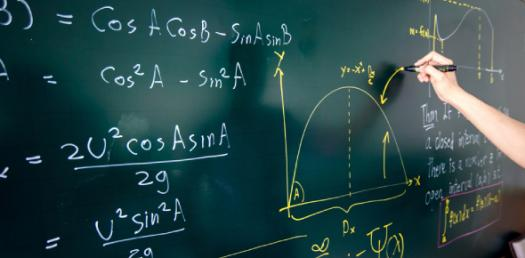# Practice Test: Math And Science Trivia Questions Quiz!

14 QuestionsSettingsWelcome to this math and science trivia questions quiz. If you are having a hard time when it comes to math and science, worry not like the quiz below is the first of many designed to help you refresh your understanding on some topics. Do give it a shot and get to see how well you will do. All the best!

Related Topics
• 1.
What is the value of 5/16 divided 5/32?
• A.

3.0

• B.

2.5

• C.

2.0

• D.

1.5

• 2.
Convert 2.5 gallons into liters.
• A.

10.365 litres

• B.

11.365 litres

• C.

12.365 litres

• D.

13.365 litres

• 3.
What is the value of the square root of 10404?
• A.

101

• B.

102

• C.

103

• D.

104

• 4.
Convert 30 cm into inches.
• A.

11.91 inch

• B.

11.81 inch

• C.

11.71 inch

• D.

11.61 inch

• 5.
What is the density of aluminum?
• A.

2.1 gm/cc

• B.

2.4 gm/cc

• C.

2.7 gm/cc

• D.

2.9 gm/cc

• 6.
The percentage of carbon content in the cast iron ranges from
• A.

0.15% to 1.5%

• B.

1.5% to 2%

• C.

2% to 4%

• D.

4% to 6%

• 7.
Malleable cast iron increases
• A.

Ductility

• B.

Malleability

• C.

Brittlens

• D.

Hardness

• 8.
Which is the purest form of iron?
• A.

Wrought iron

• B.

Cast iron

• C.

Pig iron

• D.

Steel

• 9.
Steel is produce from
• A.

Blast furnace

• B.

Cupola furnace

• C.

Bessemer converter

• D.

Puddling furnace

• 10.
High carbon steel whose carbon content range is
• A.

1.4 to 1.8%

• B.

1.3 to 1.6%

• C.

1.2 to 1.4%

• D.

1.1 to 1.4%

• 11.
Name the type of steel used to manufacture magnets?
• A.

Vandium steel

• B.

Cobalt steel

• C.

High speed steel

• D.

Nickel steel

• 12.
Bronze is an alloy of
• A.

Copper and zinc

• B.

Copper and tin

• C.

• D.

• 13.
Brass is an alloy of
• A.

Copper and zinc

• B.

Tin and zinc

• C.

Copper and tin

• D.

Copper and aluiminium

• 14.
Solder is an alloy of
• A.